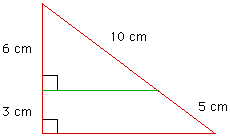Date: Tue, 06 May 1997 18:26:30 -0400
Subject: math question

I am doing the area of trapezoids and mixed polygons and I was wondering if you can help me figure out this problem.

A line segment drawn parallel to a leg of a right triangle divides the other leg into segments of 3cm and 6 cm and the hypotenuse into segments of 5cm and 10 cm. The two figures formed are a triangle and a trapezoid. Find the area of each.

I would appreciate if you would email me back the solution.

We are using the formula

A=1/2(b1 + b2)H

Thanks,
Mary

Hi Mary

As you describe the problem there are two ways to draw the diagram. The inside triangle can have a leg of length 6cm and hypotenuse of length 10cm or a leg of length 3cm and hypotenuse of length 5cm. I will describe the solution in the former case. The other situation is similar.The given triangle has a leg of length 9cm and hypotenuse of length 15cm so, using the theorem of Pythagoras, the length of the other leg is 12cm. Likewise the inside triangle is a right triangle with one side of length 6cm and hypotenuse of length 10cm so its other leg is of length 8cm. Now you know the four sides of the trapezoid and its height is 3cm so you can find its area.
Cheers,
Harley

Go to Math Central

To return to the previous page use your browser's back button.